High School Math : Simplifying Trigonometric Functions

Example Questions

Example Question #1 : Simplifying Trigonometric Functions

Simplify the function below: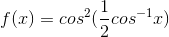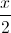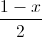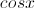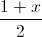Explanation:

We need to use the following formulas:

a)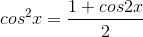and

b)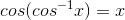We can simplifyas follows: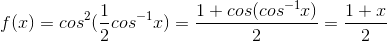All High School Math Resources